# NCERT Solutions for Class 9 Maths chapter 9 Exercise 9.2 - Areas of Parallelograms and Triangles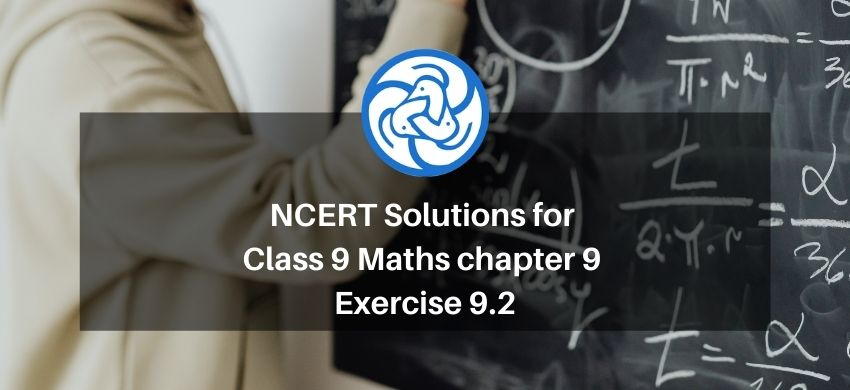Hey, are you a class 9 Student and Looking for Ways to Download NCERT Solutions for Class 9 Maths chapter 9 Exercise 9.2? If Yes then you are at the right place.

Here we have listed Class 9 maths chapter 9 exercise 9.2 solutions in PDF that is prepared by Kota’s top IITian’s Faculties by keeping Simplicity in mind.

If you want to score high in your class 9 Maths Exam then it is very important for you to have a good knowledge of all the important topics, so to learn and practice those topics you can use eSaral NCERT Solutions.

So, without wasting more time Let’s start.

### Download The PDF of NCERT Solutions for Class 9 Maths chapter 9 Exercise 9.2 "Areas of Parallelograms and Triangles"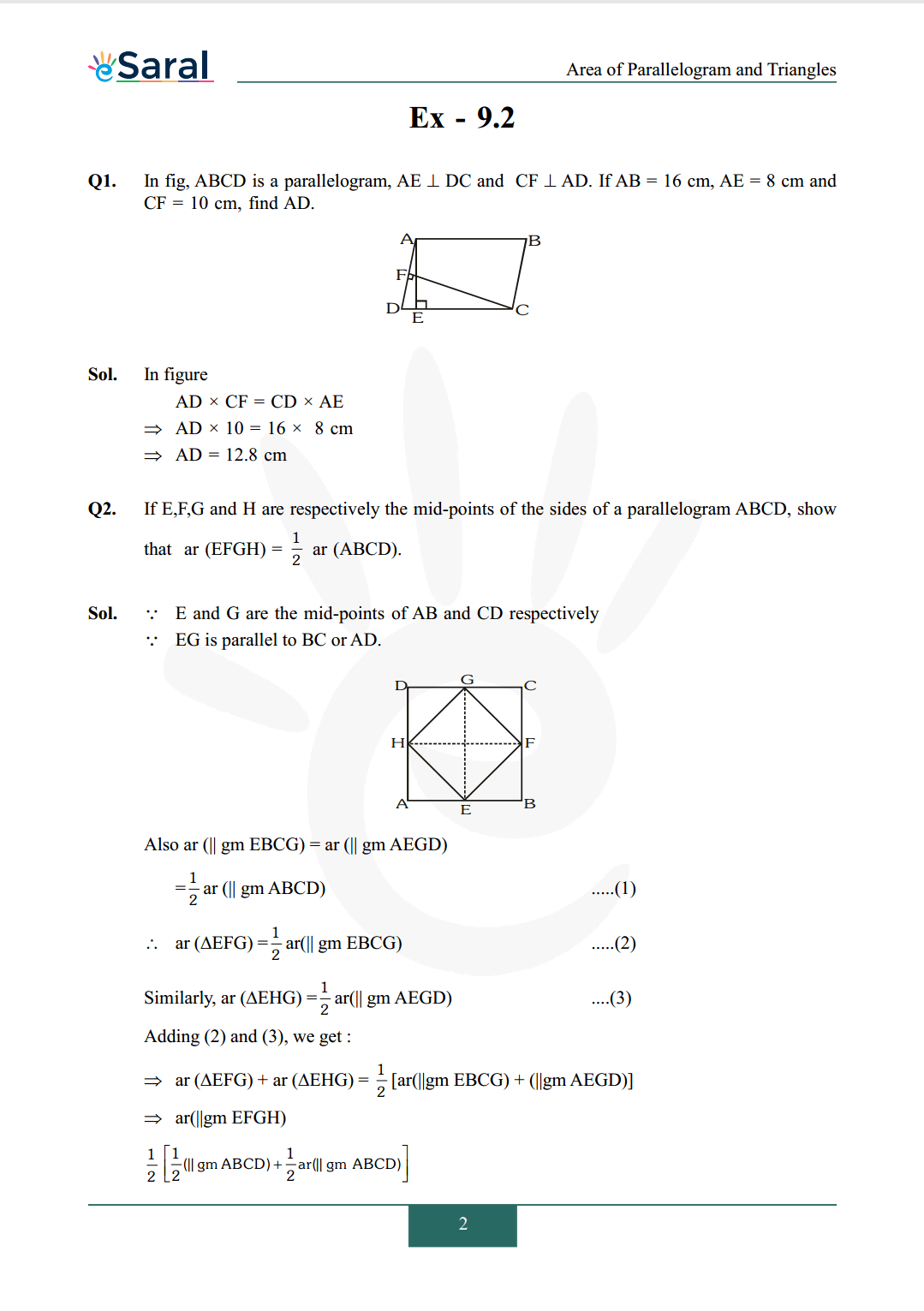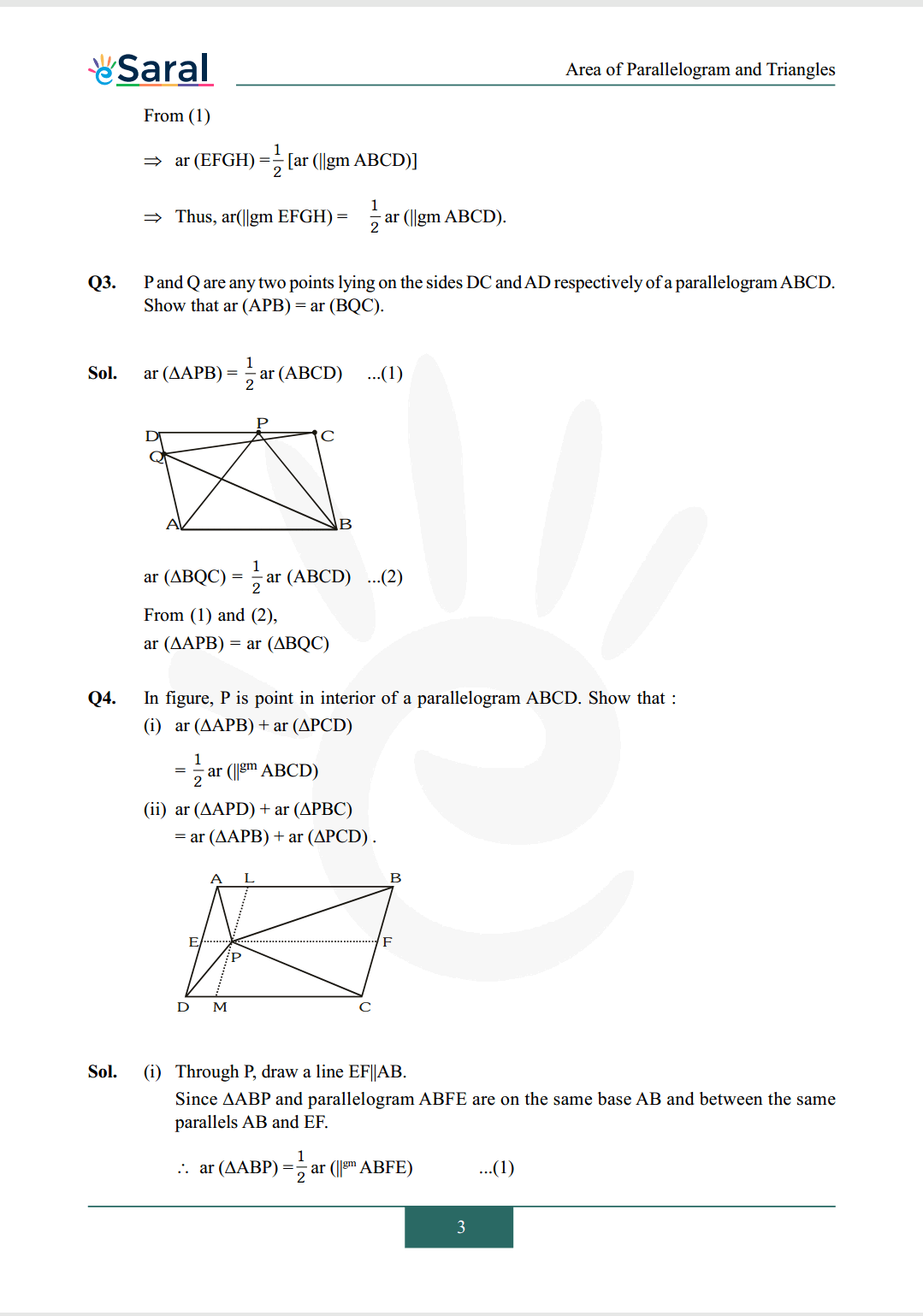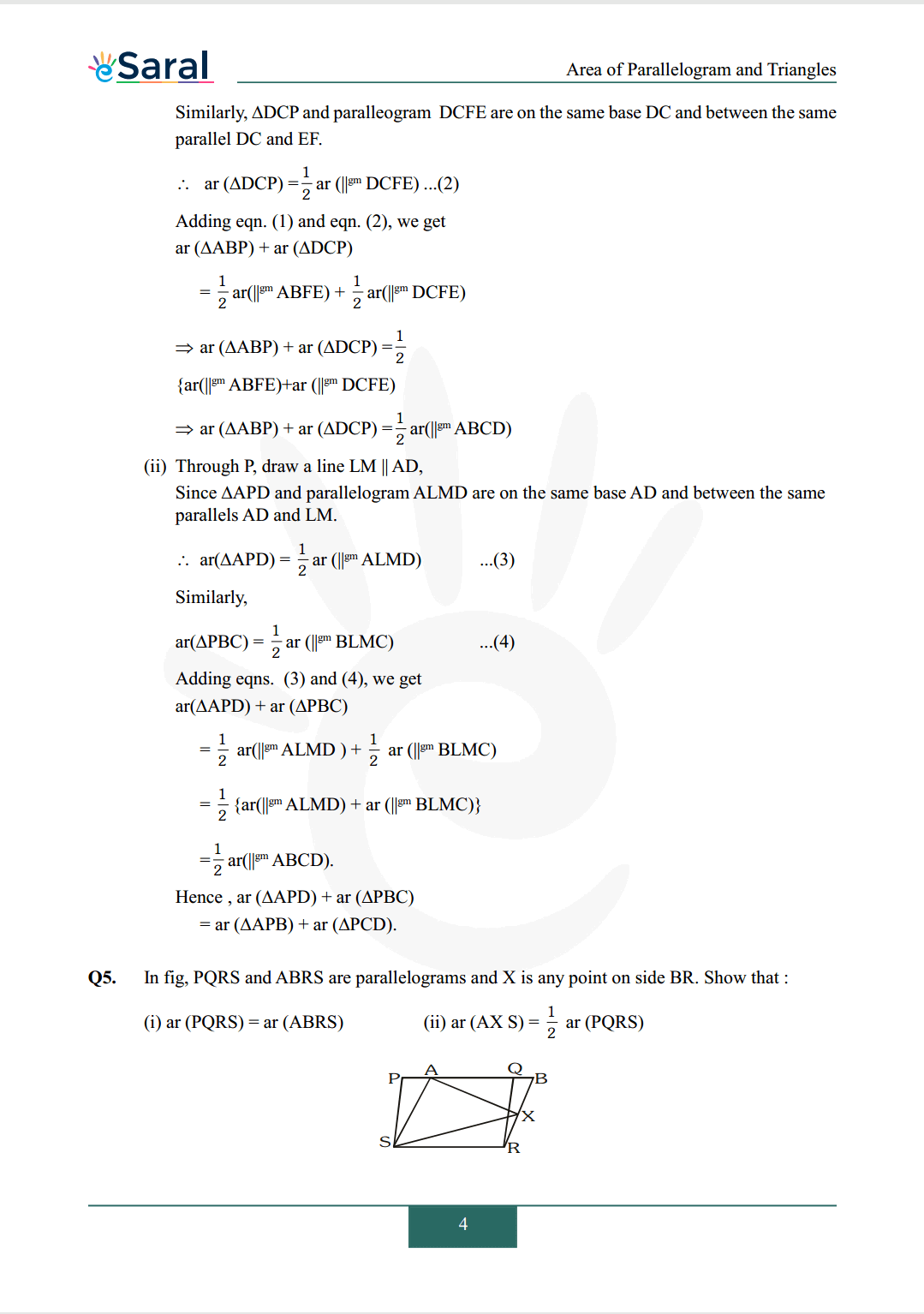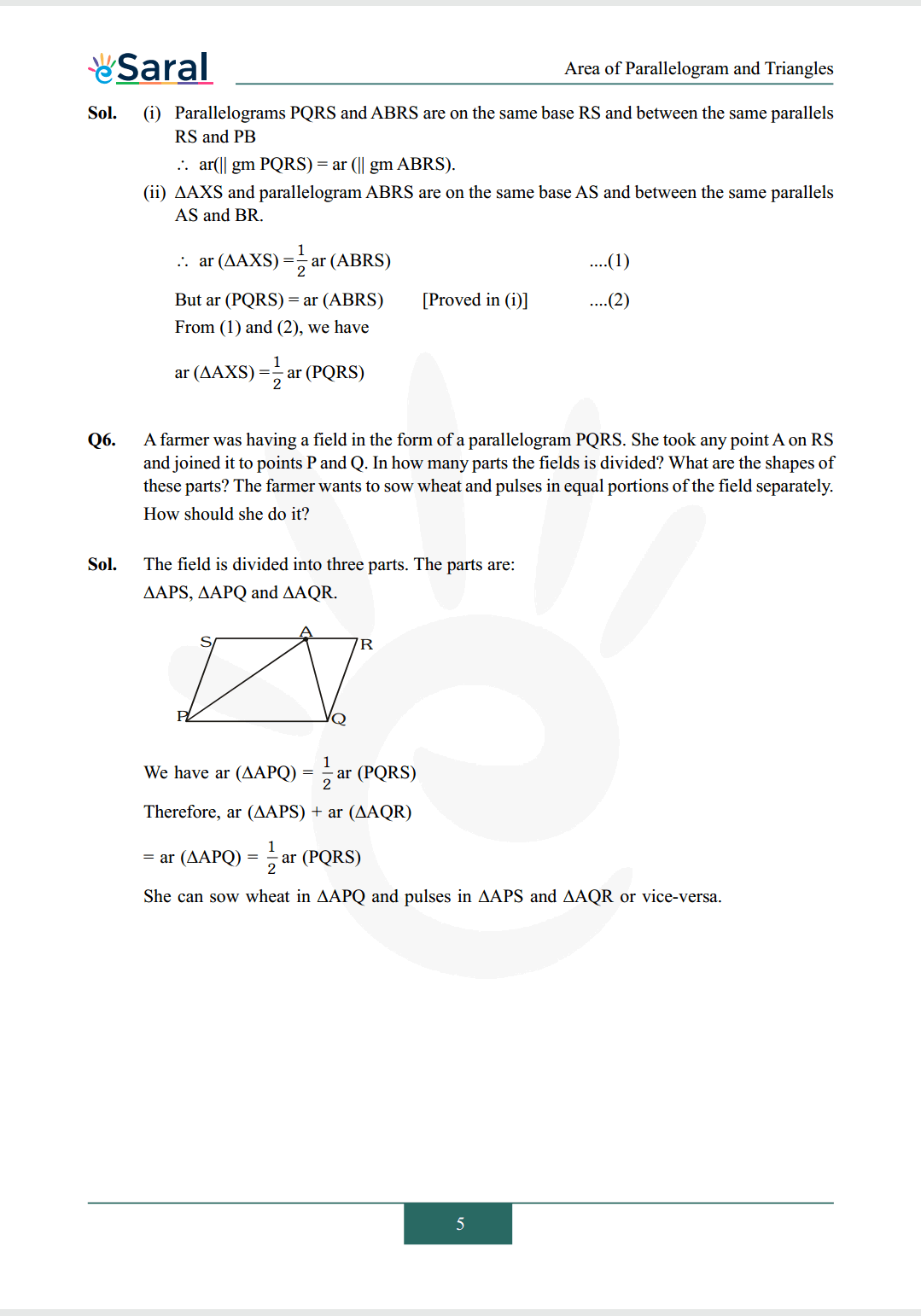Q1. In fig, $\mathrm{ABCD}$ is a parallelogram, $\mathrm{AE} \perp \mathrm{DC}$ and $\mathrm{CF} \perp \mathrm{AD}$. If $\mathrm{AB}=16 \mathrm{~cm}, \mathrm{AE}=8 \mathrm{~cm}$ and $\mathrm{CF}=10 \mathrm{~cm}$, find $\mathrm{AD}$.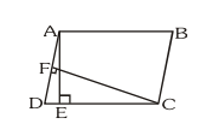Q2. If $\mathrm{E}, \mathrm{F}, \mathrm{G}$ and $\mathrm{H}$ are respectively the mid-points of the sides of a parallelogram $\mathrm{ABCD}$, show that ar (EFGH) $=\frac{1}{2}$ ar $(\mathrm{ABCD})$.

Q3. $\mathrm{P}$ and $\mathrm{Q}$ are any two points lying on the sides $\mathrm{DC}$ and $\mathrm{AD}$ respectively of a parallelogram $\mathrm{ABCD}$. Show that ar $(\mathrm{APB})=\operatorname{ar}(\mathrm{BQC})$.

Q4. In figure, $\mathrm{P}$ is point in interior of a parallelogram $\mathrm{ABCD}$. Show that:
(i) ar ( $\triangle \mathrm{APB})+\operatorname{ar}(\triangle \mathrm{PCD})$
$=\frac{1}{2} \operatorname{ar}\left(\|^{\mathrm{gm}} \mathrm{ABCD}\right)$
(ii) ar ( $\triangle \mathrm{APD})+$ ar $(\Delta \mathrm{PBC})$
$=\operatorname{ar}(\Delta \mathrm{APB})+\operatorname{ar}(\Delta \mathrm{PCD})$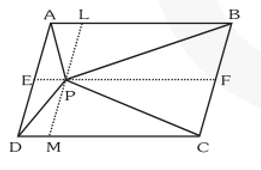Q5. In fig, PQRS and ABRS are parallelograms and $\mathrm{X}$ is any point on side BR. Show that:
(i) $\operatorname{ar}(\mathrm{PQRS})=\operatorname{ar}(\mathrm{ABRS})$
(ii) $\operatorname{ar}(\mathrm{AX} \mathrm{S})=\frac{1}{2}$ ar $(\mathrm{PQRS})$Q6. A farmer was having a field in the form of a parallelogram PQRS. She took any point A on RS and joined it to points $\mathrm{P}$ and $\mathrm{Q} .$ In how many parts the fields is divided? What are the shapes of these parts? The farmer wants to sow wheat and pulses in equal portions of the field separately. How should she do it?

Class 9 NCERT Maths Book Free PDF

Class 9 NCERT Maths Exemplar Free PDF

Complete Solutions for Class 9 Maths chapter 9

Class 9 Maths Chapter 8 Exercise 8.1 Solutions Free

Class 9 Maths Chapter 8 Exercise 8.2 Solutions Free

If you have any Confusion related to NCERT Solutions for Class 9 Maths chapter 9 Exercise 9.2 then feel free to ask in the comments section down below.

To watch Free Learning Videos on Class 9 by Kota’s top Faculties Install the eSaral App